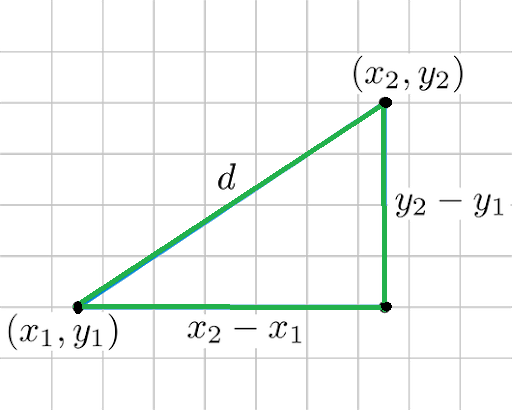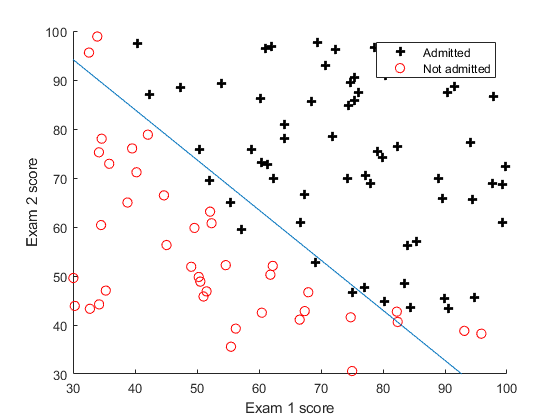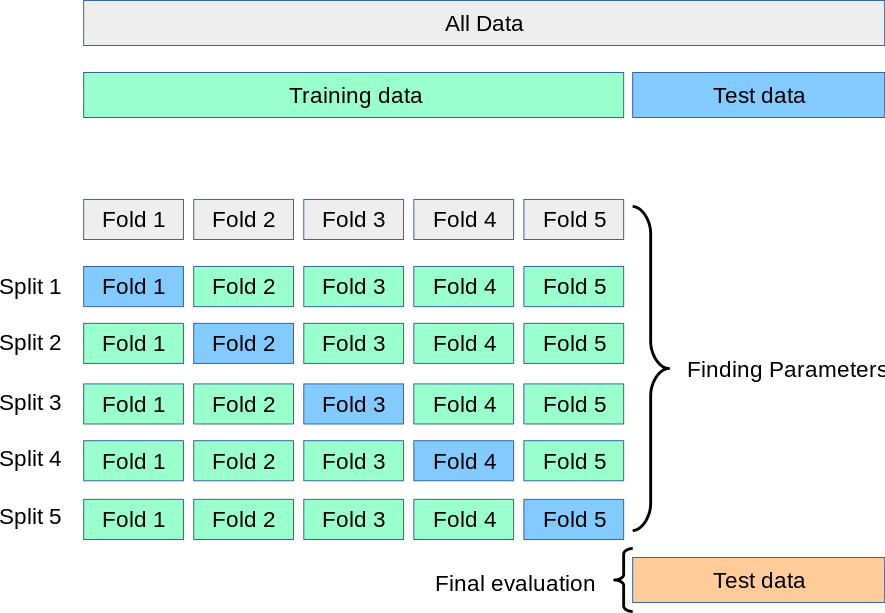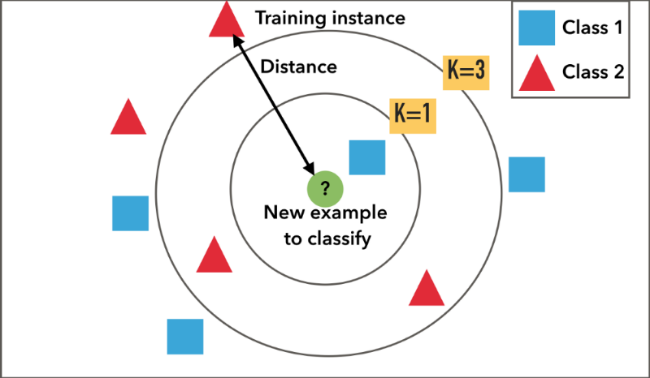# K-Nearest NeighbourK Nearest neighbour (KNN) is one of the most basic classification algorithms. It follows the assumption that similar things exist in close proximity to each other.The K-nearest neighbour algorithm calculates the distance between the various points whose category is known and then selects the shortest distance for the new data point. It is an algorithm that comes under supervised learning scenarios, which means we already have the predefined classes available.

The K in the KNN algorithm refers to the number of the neighbours considered, which can take any whole number as input.Consider an example that shows whether a certain product is normal or has an anomaly, where blue squares show the normal product, and red triangles are anomalies. Green circles are the product that we want to predict. In this case, if we consider the value of K to be 3, i.e., inner circle, the object is classified as an anomaly due to vote out by the triangle. Still, if we consider K = 5, the product is classified as normal due to most blue squares.

To conclude, we can say in simpler terms that KNN takes the nearest neighbors of the point and tries to classify it based on most of the closest points.

## HOW TO CONSIDER DISTANCES BETWEEN POINTS?

Certain distances are commonly used while using the KNN algorithm.

1. Euclidean Distance:-
Consider 2 points and so the euclidean distance between them would be. This distance denotes the shortest distance between the two points it is also known as  Norm.2. Manhattan distance:-

Manhattan distance refers to the sum of the absolute difference between the points3.Minkowski distance:-

For the distance between two points, it is calculated as

If we make p = 1, it becomes Manhattan distance, and when p = 2, it becomes euclidean distance.

## How to select the value of K?

Before we dive into understanding how to select the optimal value of K, we need to understand what decision boundary means.Consider a data set consisting of circles and pus labels so if we try to generalize the relation or the pattern of classification, we can draw a line or a curve as drawn by the blue line, which easily separates the majority of plus and the circles. The curve acts as a guide to the algorithm in classifying the point. The curve is known as the decision boundary.

Now, if we consider another data set that has points in the following distribution and considers

K = 1, then the decision boundary will be something like

And if the test point is closer to the center circle, it would be classified as a circle rather than a cross that is in the majority around the center. Such kind of model is known as an overfitted model

In another case, if we consider the value of K to be very large, we may end up classifying everything as square as the decision boundary would tend more towards the majority of the label, which being square; in this case, such a model is known as underfit model thus it is important to select the optimal value of K

Now we may ask how we can calculate perfect K.
To answer that, there is no step-by-step process to calculate the perfect value of the K.

To calculate the value of the K, we will have to go by approximation and guess and to find the best match.

Let’s understand this with an example.

 from sklearn import datasets from sklearn.neighbors import KNeighborsClassifier from sklearn.model_selection import train_test_split

This data set contains the data about whether a cancer is malignant or not.

 X_train , X_test , Y_train , Y_test = train_test_split(dataset.data , dataset.target , test_size = 0.2,random_state=0)

We then split data into training and testing datasets

 clf = KNeighborsClassifier() clf.fit(X_train, Y_train)

Next, we select the classifier as Kneigbor and fit it using X_train and Y_train data

 clf.score(X_test, Y_test)

0.9385964912280702

Upon scoring the algorithm, we get a score of .93

By default, KNN selects the value of K to be 5.

Now we may argue to change the score; we can try and set the value of K to some different number and then rerun over the test data to increase the score. Still, in reality, we are trying to fit the test data into the model, which in the end, would defeat the purpose of the test data.

As an alternative, we may say that what if we try to train the algorithm using training data and then repass it as test data to decide the optimum value of K. Still, in that case, we will end up getting a 100% accuracy over training data. Still, it will fail on the test data and give low scores.

## ValidationNow to find the optimum value of K, what we can do is take our training data and split it into two parts in a 60:40 ratio then we can use the 60% of the data to train our model and the remaining 40% of the training data to test and determine the value of K without compromising the integrity of the Test data. This process is known as Cross-validation.

However, it is also said that the more the training data is available, the better is the model, but we already lost 40% of our data to testing cases to overcome this, we use K fold cross-validation.

Consider we have a box denoting our training data.

Then k fold cross validation states that we can split our training data into P number of parts and use P -1 parts for training and the remaining 1 for testing purposes.

In simpler words, we can use 1 part at a time for testing and the remaining for training, i.e., if we considered 1st part for testing, then from 2nd part onwards up to the end will be used for training purposes, the next 2nd part would be considered for testing and remaining parts would be considered for training, so on and so forth.

After calculating the scores of various parts, we can take an average of the scores and consider it as the score for the particular value of K.

Let’s see this using an example.

Continuing on the above example where the breast cancer dataset was chosen.

Now to implement K fold CV, we use a for loop and repeatedly score the data for the various  values of K and store them in an array so it would be easier to plot using matplotlib

 x_axis = [] y_axis = [] for i in range(1, 26, 2): clf = KNeighborsClassifier(n_neighbors = i) score = cross_val_score(clf, X_train, Y_train) x_axis.append(i) y_axis.append(score.mean())

We use odd values of the K while considering the values of K assume in the neighbor of the unknown point; we get an equal number of labels A or label B it would be difficult to classify. Thus, to avoid it, we consider an odd number of neighbours.

 import matplotlib.pyplot as plt plt.plot(x_axis, y_axis) plt.show()

We import matplotlib and plot the variation of the score against the various value of K

From this plot, we come to know that the best accuracy comes near K = 7 Thus, we will consider K as 7.

Now let’s try to implement this algorithm from scratch by ourselves

Using K fold CV we came to know that we would get the best result at K = 7 so let’s run the algorithm using K = 7 and score it on the same random state using sklearn

 from sklearn import datasets from sklearn.neighbors import KNeighborsClassifier from sklearn.model_selection import train_test_split

Importing libraries and dataset from sklearn

 dataset = datasets.load_breast_cancer() X_train, X_test, Y_train, Y_test = train_test_split(dataset.data, dataset.target, test_size = 0.2, random_state = 0)

Loading dataset and splitting the data into training and testing data in an 80:20 ratio, since we would be trying to implement this algorithm ourselves, we are splitting data using a particular random state to get some split every time

 clf = KNeighborsClassifier(n_neighbors=7) clf.fit(X_train, Y_train)

Calling the classifier and specifying the value of K as 7 then we call the fit function so as to train the model

 clf.score(X_test, Y_test)

Upon scoring the algorithm, we get a score of

0.94736842105263153

## Limitation of KNNKNN’s limitations: KNN is an extremely strong algorithm. It is also known as a “slow learner.” It does, however, have the following limitations:

1. Doesn’t function well with huge datasets: Because KNN is a distance-based method, the cost of computing the distance between a new point and each old point is quite high, degrading the algorithm’s speed.
2. Doesn’t function well with many dimensions: Same as above. The cost of calculating distance increases in higher dimensional space, affecting performance.
3. Sensitive to outliers and missing data: Because KNN is sensitive to outliers and missing values, we must first impute missing values and remove outliers before using the KNN method.

K-Nearest Neighbors (K-NN) is a popular algorithm used in machine learning for both classification and regression tasks. It is a non-parametric method that determines the classification or prediction of a new data point based on the majority vote or average of its k nearest neighbors in the feature space.

### The algorithm works as follows:

1. Dataset Preparation: To apply the K-NN algorithm, the dataset needs to be prepared. It should include labeled data points, where each data point has a set of features and a corresponding class or target variable. It is important to normalize or standardize the features to ensure that no single feature dominates the distance calculations.
2. Determining the Value of k: The value of k, the number of neighbors to consider, needs to be determined. It is a hyperparameter that can be chosen through trial and error or using techniques such as cross-validation to find the optimal value for the specific problem. A small k value might lead to overfitting, while a large k value may lead to oversimplification.
3. Calculating Distances: For a new data point, the algorithm calculates the distances between the data point and all other data points in the training set using a distance metric such as Euclidean distance, Manhattan distance, or Minkowski distance. The distance metric chosen depends on the nature of the data and the problem at hand.
4. Identifying the Neighbors: The k nearest neighbors of the new data point are identified based on the calculated distances. These neighbors are the data points with the shortest distances to the new data point.
5. Classification or Regression: For classification tasks, the class label of the new data point is determined based on the majority class among its k nearest neighbors. Each neighbor’s class is given equal weight in the voting process. In the case of regression tasks, the predicted value of the new data point is determined by taking the average of the target variable values of its k nearest neighbors.
6. Handling Ties: In situations where there is a tie in the majority voting for classification or the average value for regression, techniques such as weighted voting or considering additional neighbors can be used to break the tie and make a final prediction.